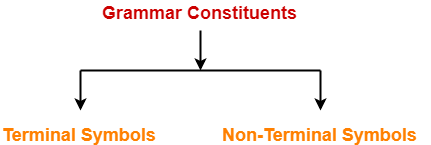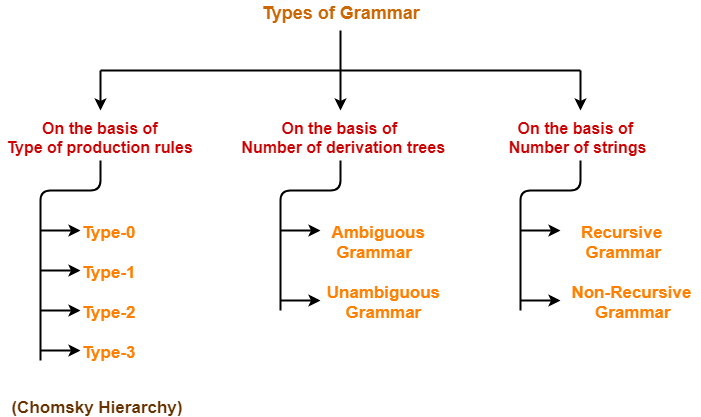# Grammar in Automata | Types of Grammar

## Grammar in Automata-

### Formal Definition-

A Grammar is a 4-tuple such that-

G = (V , T , P , S)

where-

• V = Finite non-empty set of non-terminal symbols
• T = Finite set of terminal symbols
• P = Finite non-empty set of production rules
• S = Start symbol

## Grammar Constituents-

A Grammar is mainly composed of two basic elements-1. Terminal symbols

2. Non-terminal symbols

### 1. Terminal Symbols-

• Terminal symbols are those which are the constituents of the sentence generated using a grammar.
• Terminal symbols are denoted by using small case letters such as a, b, c etc.

### 2. Non-Terminal Symbols-

• Non-Terminal symbols are those which take part in the generation of the sentence but are not part of it.
• Non-Terminal symbols are also called as auxiliary symbols or variables.
• Non-Terminal symbols are denoted by using capital letters such as A, B, C etc.

## Examples of Grammar-

### Example-01:

Consider a grammar G = (V , T , P , S) where-

• V = { S } // Set of Non-Terminal symbols
• T = { a , b } // Set of Terminal symbols
• P = { S → aSbS , S → bSaS , S → ∈ } // Set of production rules
• S = { S } // Start symbol

This grammar generates the strings having equal number of a’s and b’s

### Example-02:

Consider a grammar G = (V , T , P , S) where-

• V = { S , A , B } // Set of Non-Terminal symbols
• T = { a , b } // Set of Terminal symbols
• P = { S → ABa , A → BB , B → ab , AA → b } // Set of production rules
• S = { S } // Start symbol

## Types of Grammars-

Grammars are classified on different basis as-We will discuss all these types of grammar one by one in detail.

## Equivalent Grammars-

 Two grammars are said to be equivalent if they generate the same languages.

Also Read- Language of Grammar

### Example-

Consider the following two grammars-

S → aSb / ∈

#### Grammar G2-

S → aAb / ∈

A → aAb / ∈

Both these grammars generate the same language given as-

L = { anbn , n>=0 }

Thus, L(G1) = L(G2)

Since both the grammars generate the same language, therefore they are equivalent.

 ∴ G1 ≡ G2

To gain better understanding about Grammars in Automata,

Watch this Video Lecture

Next Article- Ambiguous Grammar

Get more notes and other study material of Theory of Automata and Computation.

Watch video lectures by visiting our YouTube channel LearnVidFun.

SummaryArticle Name
Grammar in Automata | Types of Grammar
Description
In automata, Grammar is defined as 4-tuple G (V, T, P, S). Example of Grammar. Types of Grammar- Ambiguous and Unambiguous Grammar, Recursive and Non-Recursive Grammar, Chomsky Hierarchy.
Author
Publisher Name
Gate Vidyalay
Publisher Logo